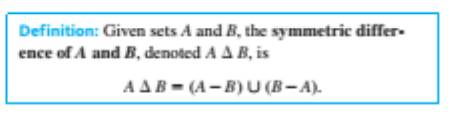Chapter 6.3, Problem 46ES### Discrete Mathematics With Applicat...

5th Edition
EPP + 1 other
ISBN: 9781337694193

#### Solutions

Chapter
Section### Discrete Mathematics With Applicat...

5th Edition
EPP + 1 other
ISBN: 9781337694193
Textbook Problem
10 views

#Let A = { 1 , 2 , 3 , 4 } , B = { 3 , 4 , 5 , 6 } , and C = { 5 , 6 , 7 , 8 } Find each of the following sets:  a.  A Δ B  b.  B Δ C  c.  A Δ C  d.  ( A Δ B ) Δ C

To determine

(a)

To derive:

Find

AΔB

Explanation

Given information

A={1,2,3,4},B={3,4,5,6}

Concept used:

AΔB={x/x is an element in A, and x is not in B}{x is an element in B,but not an element of A}

Calculation:

A={1,2,3,4},B={3,4,

To determine

(b)

BΔC

To determine

(c)

AΔC

To determine

(d)

(AΔB)ΔC

### Still sussing out bartleby?

Check out a sample textbook solution.

See a sample solution

#### The Solution to Your Study Problems

Bartleby provides explanations to thousands of textbook problems written by our experts, many with advanced degrees!

Get Started